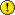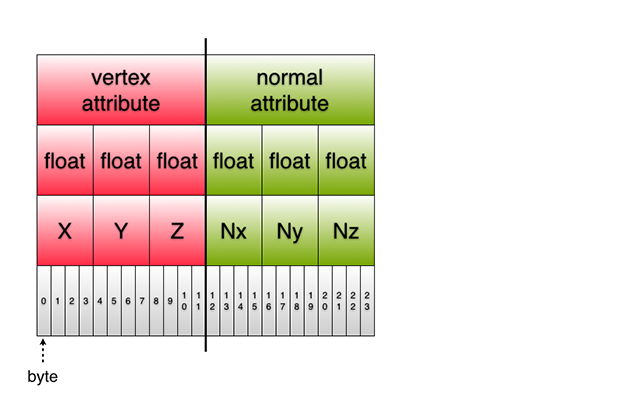# RhinoMobile - Rendering PipelineWarning: This wiki entry is a work-in-progress. Information may be missing or incomplete.

## Vertex Buffer Objects (VBOs)

The Vertices

The vertices of our DisplayMesh are fed to the GPU (via a shader) as an object called a Vertex Buffer Object.

This is how a (hypothetical) Vertex-only VBO might look in code:

```public struct VData {  //This is a redundant struct, but it's for example purposes only
public Point3f Vertex;
}```

We say hypothetical because it would be redundant to define this as a struct. Also, we don't use a Vertex only VBO in our code, but it's a good place to start because it illustrates some points about VBOs and how to construct them.

It's important to be aware of our memory footprint and the sizes of each type as we pack them into a VBO. So, here are the C# value types we are using the construct our VBOs:

• float = 32 bits, 4 bytes
• short = 16 bits, 2 bytes
• ushort = 16 bits, 2 bytes

This is a diagram of how a simple, vertex only Vertex Buffer Object would look like in memory:Each item in a VBO is called an attribute. Notice the attribute separator in the diagram above. This separator would device the first vertex from the second. The vertex is made up of 3 floats (X,Y,Z) at 4 bytes each, for a total of 12 bytes.

Adding a normal vector

```public struct VNData  {
public Point3f Vertex;
public Vector3f Normal;
}```

This is a diagram of how an interleaved Vertex and Color VBO would look like in memory:Color too!

We define a simple struct for our VertexColor VBO in code like this:

```public struct VCData {
public Point3f Vertex;
public Color4f Color;
}```

Indexing the vertices*

`public ushort[] IndexData { get; private set; }`

## Frame Buffer Objects (VBOs)

COMING SOON…

developer/rhinomobile/rendering_pipeline.txt · Last modified: 2020/08/14 (external edit)

### Page Tools